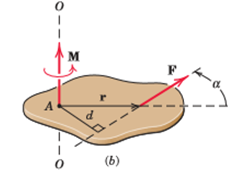Courses

# Moment of a Force about a Point Mechanical Engineering Notes | EduRev

## Mechanical Engineering : Moment of a Force about a Point Mechanical Engineering Notes | EduRev

The document Moment of a Force about a Point Mechanical Engineering Notes | EduRev is a part of the Mechanical Engineering Course Engineering Mechanics - Notes, Videos, MCQs & PPTs.
All you need of Mechanical Engineering at this link: Mechanical Engineering

Moment of Force about a Point

The moment of a force is only defined with respect to a certain point P (it is said to be the "moment about P"), and in general when P is changed, the moment changes. However, the moment (torque) of a couple is independent of the reference point P: Any point will give the same moment. In other words, a torque vector, unlike any other moment vector, is a "free vector".

(This fact is called Varignon's Second Moment Theorem.)

The proof of this claim is as follows: Suppose there are a set of force vectors F1, F2, etc. that form a couple, with position vectors (about some origin P) r1, r2, etc., respectively. The moment about P is

M = r* F+ r2* F+ r3* F+ ..

Now we pick a new reference point P' that differs from P by the vector r. The new moment is

M’ = (r1 + r)x F+ (r2 + r)x F+ (r3 + r)x F+ ...

Now the distributive property of the cross product implies

M’ = (rx F+ rx F+ rx F3+ ….) + r x (F+ F+F3 + ….)However, the definition of a force couple means that

F+ F+F3 +...= 0

Therefore,

M’ = rx F+ rx F+ rx F3+ .. =M

This proves that the moment is independent of reference point, which is proof that a couple is a free vector.

Offer running on EduRev: Apply code STAYHOME200 to get INR 200 off on our premium plan EduRev Infinity!

,

,

,

,

,

,

,

,

,

,

,

,

,

,

,

,

,

,

,

,

,

;